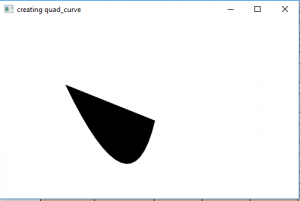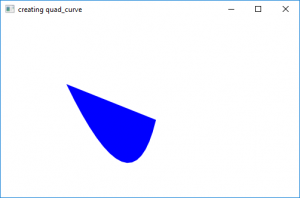# JavaFX | QuadCurve with examples

• Last Updated : 31 Aug, 2018

QuadCurve is a part of JavaFX. The Quadcurve class defines a quadratic Bézier parametric curve segment in (x, y) coordinate space. The curve passes through the starting point and the ending point and also the control point. The specified control point is used as Bézier control point.

Constructor for the class are:

2. QuadCurve(double sX, double sY, double cX, double cY, double eX, double eY):creates a new instance of quad curve with specified starting point, ending point and control point.

commonly used methods

MethodExplanation
getControlX()returns the x coordinate for the control point
getControlY()returns the y coordinate for the control point
getEndX()returns the x coordinate for the end point
getEndY()returns the y coordinate for the end point
getStartX()returns the x coordinate for the start point
getStartY()returns the y coordinate for the start point
setControlX(double value)sets the x coordinate for the control point
setControlY(double value)sets the y coordinate for the control point
setEndX(double value)sets the x coordinate for the end point
setEndY(double value)sets the y coordinate for the end point
setStartX(double value)sets the x coordinate for the start point
setStartY(double value)sets the y coordinate for the start point

The following programs will illustrate the use of QuadCurve

Java program to create a quad curve
This program creates a QuadCurve indicated by the name quad_curve( control point, start point and end point is passed as arguments).
The QuadCurve will be created inside a scene, which in turn will be hosted inside a stage. The function setTitle() is used to provide title to the stage. Then a Group is created, and the quad_curve is attached. The group is attached to the scene. Finally, the show() method is called to display the final results.

 `// Java program to create a quad curve``import` `javafx.application.Application;``import` `javafx.scene.Scene;``import` `javafx.scene.shape.DrawMode;``import` `javafx.scene.layout.*;``import` `javafx.event.ActionEvent;``import` `javafx.scene.shape.QuadCurve;``import` `javafx.scene.control.*;``import` `javafx.stage.Stage;``import` `javafx.scene.Group;``public` `class` `quad_curve_0 ``extends` `Application {`` ` `    ``// launch the application``    ``public` `void` `start(Stage stage)``    ``{``        ``// set title for the stage``        ``stage.setTitle(``"creating quad_curve"``);`` ` `        ``// create a quad_curve``        ``QuadCurve quad_curve = ``new` `QuadCurve(``10``.0f, ``10``.0f, ``120``.0f, ``240``.0f, ``160``.0f, ``70``.0f);`` ` `        ``// create a Group``        ``Group group = ``new` `Group(quad_curve);`` ` `        ``// translate the quad_curve to a position``        ``quad_curve.setTranslateX(``100``);``        ``quad_curve.setTranslateY(``100``);`` ` `        ``// create a scene``        ``Scene scene = ``new` `Scene(group, ``500``, ``300``);`` ` `        ``// set the scene``        ``stage.setScene(scene);`` ` `        ``stage.show();``    ``}`` ` `    ``public` `static` `void` `main(String args[])``    ``{``        ``// launch the application``        ``launch(args);``    ``}``}`

Output
:Java program to create a quad curve and set a fill for quad curve

This program creates a QuadCurve indicated by the name quad_curve( control point, start point and end point are set using setControlX(), setControlY(), setStartX(), setStartY(), setEndX(), ans setEndY() functions). The QuadCurvewill be created inside a scene, which in turn will be hosted inside a stage. The function setTitle() is used to provide title to the stage. Then a Group is created, and the quad_curve is attached.The group is attached to the scene. Finally, the show() method is called to display the final results.The function setFill() is used to set fill for the quad curve.

 `// Java program to create a quad curve``// and set a fill for quad curve``import` `javafx.application.Application;``import` `javafx.scene.Scene;``import` `javafx.scene.shape.DrawMode;``import` `javafx.scene.layout.*;``import` `javafx.event.ActionEvent;``import` `javafx.scene.shape.QuadCurve;``import` `javafx.scene.control.*;``import` `javafx.stage.Stage;``import` `javafx.scene.Group;``import` `javafx.scene.paint.Color;``public` `class` `quad_curve_1 ``extends` `Application {`` ` `    ``// launch the application``    ``public` `void` `start(Stage stage)``    ``{``        ``// set title for the stage``        ``stage.setTitle(``"creating quad_curve"``);`` ` `        ``// create a quad_curve``        ``QuadCurve quad_curve = ``new` `QuadCurve();`` ` `        ``// set start``        ``quad_curve.setStartX(``10``.0f);``        ``quad_curve.setStartY(``10``.0f);`` ` `        ``// set control coordinates``        ``quad_curve.setControlX(``120``.0f);``        ``quad_curve.setControlY(``240``.0f);`` ` `        ``// set end coordinates``        ``quad_curve.setEndX(``160``.0f);``        ``quad_curve.setEndY(``70``.0f);`` ` `        ``// create a Group``        ``Group group = ``new` `Group(quad_curve);`` ` `        ``// translate the quad_curve to a position``        ``quad_curve.setTranslateX(``100``);``        ``quad_curve.setTranslateY(``100``);`` ` `        ``// set fill for the quad curve``        ``quad_curve.setFill(Color.BLUE);`` ` `        ``// create a scene``        ``Scene scene = ``new` `Scene(group, ``500``, ``300``);`` ` `        ``// set the scene``        ``stage.setScene(scene);`` ` `        ``stage.show();``    ``}`` ` `    ``public` `static` `void` `main(String args[])``    ``{``        ``// launch the application``        ``launch(args);``    ``}``}`

output:Note : The above programs might not run in an online IDE please use an offline compiler

Reference: